﻿ Molar Mass

#Molar Mass Conversion Factors

 Molar Mass for Elements The mass in grams of one mole of substance is called molar mass. Each element has its own unique molar mass. For example, carbon’s molar mass is 12.011 g/mol, and magnesium’s molar mass is 24.3050 g/mol. To see why these elements have different molar masses, we need to remember that the atoms of different elements contain different numbers of protons, neutrons, and electrons, so they have different masses. The atomic masses given in the periodic table inside the front cover of this book represent the different weighted average masses of the naturally occurring atoms of each element. Different atomic masses lead to different molar masses. For example, the atomic mass of magnesium (24.3050) shows us that the average mass of magnesium atoms is about twice the average mass of carbon atoms (12.011), so the mass of 6.022 x 1023 magnesium atoms (the number of atoms in 1 mole of magnesium) is about twice the mass of 6.022 x 1023 carbon atoms (the number of atoms in 1 mole of carbon). Thus the molar mass of magnesium is 24.3050 g/mol, compared to carbon’s molar mass of 12.011 g/mol.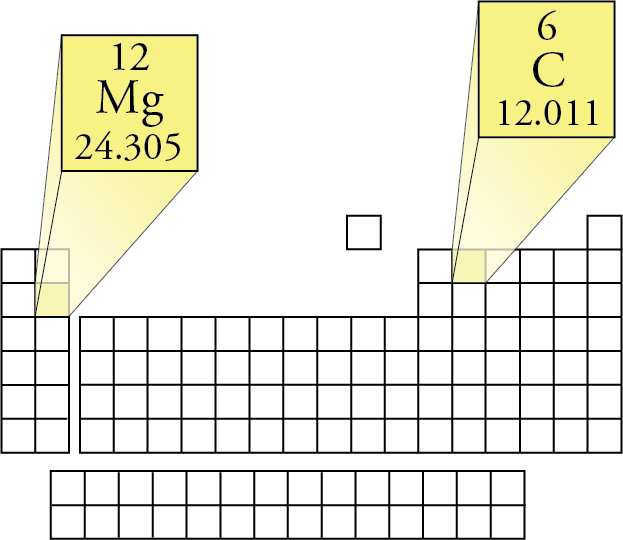The number of grams in the molar mass of an element is the same as the atomic mass. Translating atomic masses into molar masses, you can construct conversion factors that convert between the mass of an element and the number of moles of the element.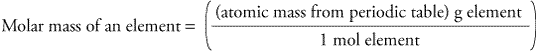For example, the atomic mass of the element neon listed on the periodic table on the inside cover of this book is 20.1797, giving a molar mass of 20.1797 g/mol. This measurement provides the following conversion factors for converting between grams and moles of neon.Lithium’s atomic mass is 6.941, so the conversion factors for converting between mass and moles of lithium areMolar Mass for Molecular Compounds The first step in the determination of the molar mass of a molecular compound is to determine the molecular mass of the compound, which is the weighted average mass of the compound’s naturally occurring molecules. This is found by adding the atomic masses of the atoms in each molecule. Molecular mass = the sum of the atomic masses of each atom in the molecule Therefore, the molecular mass of water, H2O, is equal to the sum of the atomic masses of two hydrogen atoms and one oxygen atom, which can be found on the periodic table. Molecular mass H2O                 =  2(1.00794)  +  15.9994  =  18.0153 Notice that the atomic mass of each element is multiplied by the number of atoms of that element in a molecule of the compound. The number of grams in the molar mass (grams per mole) of a molecular compound is the same as its molecular mass.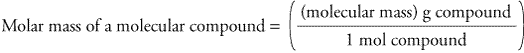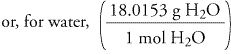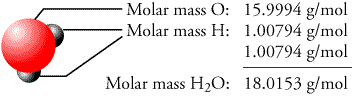Molar Mass for Ionic Compounds The first step in the determination of the molar mass of an ionic compound is to determine its formula mass, which is the weighted average of the mass of the naturally occurring formula units of the substance. (It is analogous to the atomic mass for an element and the molecular mass for a molecular substance.) Formula mass  =  the sum of the atomic masses of each atom in a formula unit The formula mass of sodium chloride is equal to the sum of the atomic masses of sodium and chlorine, which can be found on the periodic table. Formula mass NaCl                        =  22.9898  + 35.4527  =  58.4425 The number of grams in the molar mass (grams per mole) of any ionic compound is the same as its formula mass.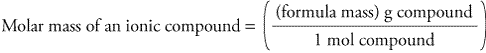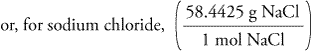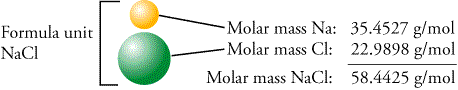Chiral Publishing Company